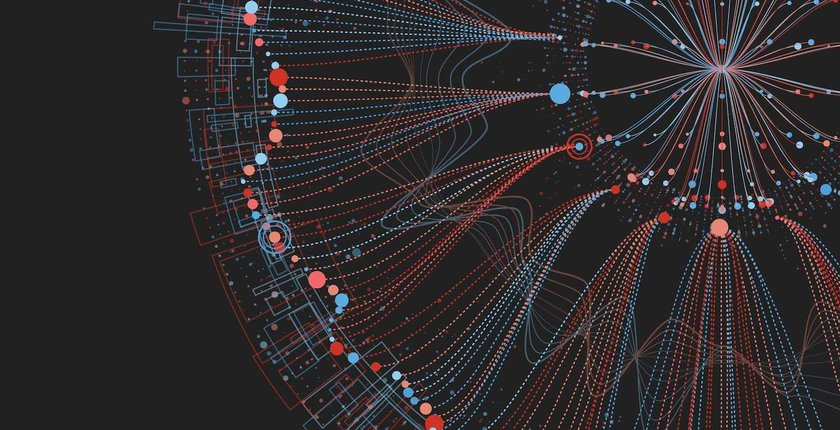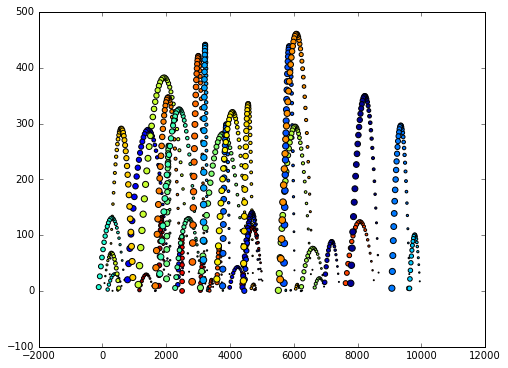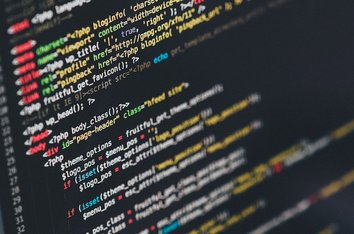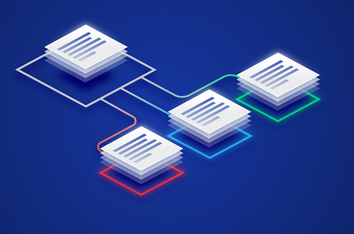Deep Learning
July 26, 2020

# DeepGraph Python implementation

DeepGraph is an open-source Python implementation of a new network representation introduced here. Its purpose is to facilitate data analysis by interpreting data in terms of network theory.

The basis of this software package is Pandas, a fast and flexible data analysis tool for the Python programming language. Utilizing one of its primary data structures, the DataFrame, we represent objects (i.e. the nodes of a network) by one DataFrame, and their pairwise relations (i.e. the edges of a system) by another DataFrame.

One of the main features of DeepGraph is an efficient and scalable creation of edges. Given a set of nodes in the form of a DataFrame (or an on disc HDFStore), DeepGraph’s core class provides methods to iteratively compute pairwise relations between the nodes (e.g. similarity/distance measures) using arbitrary, user-defined functions on the nodes’ features. These methods provide arguments to parallelize the computation and control memory consumption, making them suitable for huge data-sets and adjustable to whatever hardware you have at hand (from netbooks to cluster architectures).

Furthermore, once a graph is constructed, DeepGraph allows you to partition its nodesedges, or the entire graph by the graph’s properties and labels, enabling the aggregation, computation, and allocation of information on and between arbitrary groups of nodes. These methods also let you express elaborate queries on the information contained in a deep graph.

DeepGraph is not meant to replace or compete with already existing Python network libraries, such as NetworkX or graph_tool, but rather to combine and extend their capabilities with Pandas’ merits. The core class of DeepGraph provides interfacing methods to convert to common network representations and graph objects of popular Python network packages.

Deepgraph also implements several useful plotting methods, including drawings on geographical map projections.

It’s also possible to represent multilayer networks by deep graphs. We’re thinking of implementing an interface to a suitable package dedicated to the analysis of multilayer networks.

## Installation

DeepGraph can be installed via pip from PyPI

``pip install deepgraph``

## Requirements

The easiest way to get Python and the required/optional packages is to use Conda (or Miniconda), a cross-platform (Linux, Mac OS X, Windows) Python distribution for data analytics and scientific computing.

### Python

To use DeepGraph you need Python 2.7, 3.4 or later.

### Pandas

Pandas is an open source, BSD-licensed library providing high-performance, easy-to-use data structures and data analysis tools for the Python programming language.

Pandas is the core dependency of DeepGraph, and it is highly recommended to install the recommended and optional dependencies of Pandas as well.

### NumPy

NumPy is the fundamental package for scientific computing with Python.

Needed for internal operations.

## Recommended Packages

The following are recommended packages that DeepGraph can use to provide additional functionality.

### Matplotlib

Matplotlib is a python 2D plotting library which produces publication quality figures in a variety of hardcopy formats and interactive environments across platforms.

Allows you to use the plotting methods of DeepGraph.

### Matplotlib Basemap Toolkit

basemap is an add-on toolkit for matplotlib that lets you plot data on map projections with coastlines, lakes, rivers and political boundaries. See the basemap tutorial for documentation and examples of what it can do.

Used by plot_map and plot_map_generator to plot networks on map projections.

### PyTables

PyTables is a package for managing hierarchical datasets and designed to efficiently and easily cope with extremely large amounts of data.

Necessary for HDF5-based storage of pandas DataFrames. DeepGraph’s core class may be initialized with a HDFStore containing a node table in order to iteratively create edges directly from disc (see create_edges and create_edges_ft).

### SciPy

SciPy is a Python-based ecosystem of open-source software for mathematics, science, and engineering.

Allows you to convert from DeepGraph’s network representation to sparse adjacency matrices (see return_cs_graph).

### NetworkX

NetworkX is a Python language software package for the creation, manipulation, and study of the structure, dynamics, and functions of complex networks.

Allows you to convert from DeepGraph’s network representation to NetworkX’s network representation (see return_nx_graph).

### Graph-Tool

graph_tool is an efficient Python module for manipulation and statistical analysis of graphs (a.k.a. networks).

## Tutorials

This is a short introduction to DeepGraph. In the following, we demonstrate DeepGraph’s core functionalities by a toy data-set, “flying balls”.

First of all, we need to import some packages

``````# for plots
import matplotlib.pyplot as plt

# the usual
import numpy as np
import pandas as pd

import deepgraph as dg

# notebook display
%matplotlib inline
plt.rcParams['figure.figsize'] = 8, 6
pd.options.display.max_rows = 10
pd.set_option('expand_frame_repr', False)``````

Then, we need data in the form of a pandas DataFrame, representing the nodes of our graph

``````v = pd.read_csv('flying_balls.csv', index_col=0)
print(v)``````
``````time            x          y  ball_id
0        0  1692.000000   0.000000        0
1        0  8681.000000   0.000000        1
2        0   490.000000   0.000000        2
3        0  7439.000000   0.000000        3
4        0  4998.000000   0.000000        4
...    ...          ...        ...      ...
1163    45  2812.552734  16.503178       39
1164    46  5686.915998  14.161693       10
1165    46  3161.729086  19.381823       14
1166    46  5594.233413  57.701712       37
1167    47  5572.216748  20.588750       37

[1168 rows x 4 columns]``````

The data consists of 1168 space-time measurements of 50 different toy balls in two-dimensional space. Each space-time measurement (i.e. row of v) represents a node.

Let’s plot the data such that each ball has it’s own color

``plt.scatter(v.x, v.y, s=v.time, c=v.ball_id)``## Creating Edges

In order to create edges between these nodes, we now initiate a dg.DeepGraph instance

``````g = dg.DeepGraph(v)
g``````
``<DeepGraph object, with n=1168 node(s) and m=0 edge(s) at 0x7facf3b35dd8>``

and use it to create edges between the nodes given by g.v. For that matter, we may define a connector function

``````def x_dist(x_s, x_t):
dx = x_t - x_s
return dx``````

and pass it to g.create_edges in order to compute the distance in the x-coordinate of each pair of nodes

``````g.create_edges(connectors=x_dist)
g``````
``<DeepGraph object, with n=1168 node(s) and m=681528 edge(s) at 0x7facf3b35dd8>``
``print(g.e)``
``````dx
s    t
0    1     6989.000000
2    -1202.000000
3     5747.000000
4     3306.000000
5     2812.000000
...                ...
1164 1166   -92.682585
1167  -114.699250
1165 1166  2432.504327
1167  2410.487662
1166 1167   -22.016665

[681528 rows x 1 columns]``````

Let’s say we’re only interested in creating edges between nodes with a x-distance smaller than 1000. Then we may additionally define a selector

``````def x_dist_selector(dx, sources, targets):
dxa = np.abs(dx)
sources = sources[dxa <= 1000]
targets = targets[dxa <= 1000]
return sources, targets``````

and pass both the connector and selector to g.create_edges

``````g.create_edges(connectors=x_dist, selectors=x_dist_selector)
g``````
``<DeepGraph object, with n=1168 node(s) and m=156938 edge(s) at 0x7facf3b35dd8>``
``print(g.e)``
``````dx
s    t
0    6     416.000000
7     848.000000
19   -973.000000
24    437.000000
38    778.000000
...               ...
1162 1167  -44.033330
1163 1165  349.176351
1164 1166  -92.682585
1167 -114.699250
1166 1167  -22.016665

[156938 rows x 1 columns]``````

There is, however, a much more efficient way of creating edges that involve a simple distance threshold.

* indicates required

### More great articles### Voice Recognition for accessibility: making your website more inclusive

Voice recognition technology has the potential to make websites more accessible to individuals with disabilities by allowing them to interact with the website through voice commands.### Transfer learning and fine-tuning in Keras and Tensorflow to build an image recognition system and classify any object

This post will show you how to use transfer learning and fine-tuning to identify any customizable object categories! To recapitulate, here is the blog post series we’ll be following.